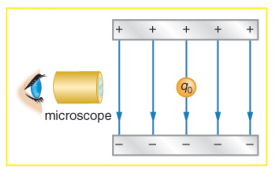×
Get Full Access to College Physics - 1 Edition - Chapter 18 - Problem 62pe
Get Full Access to College Physics - 1 Edition - Chapter 18 - Problem 62pe

×

# Integrated ConceptsThe classic Millikan oil dropISBN: 9781938168000 42

## Solution for problem 62PE Chapter 18

College Physics | 1st Edition

• Textbook Solutions
• 2901 Step-by-step solutions solved by professors and subject experts
• Get 24/7 help from StudySoup virtual teaching assistantsCollege Physics | 1st Edition

4 5 1 250 Reviews
14
1
Problem 62PE

Integrated Concepts

The classic Millikan oil drop experiment was the first to obtain an accurate measurement of the charge on an electron. In it, oil drops were suspended against the gravitational force by a vertical electric field. (See Figure 18.58.) Given the oil drop to be 1.00 μm in radius and have a

density of 920 kg/m3 : (a) Find the weight of the drop. (b) If the drop has a single excess electron, find the electric field strength needed to balance its weight.Figure 18.58 In the Millikan oil drop experiment, small drops can be suspended in an

electric field by the force exerted on a single excess electron. Classically, this experiment was used to determine the electron charge qe by measuring the electric field and mass of the drop.

Step-by-Step Solution:
Step 1 of 3

Solution  62PE

.

= 2.36x105 N/C

Step 2 of 3

Step 3 of 3

##### ISBN: 9781938168000

The answer to “?Integrated ConceptsThe classic Millikan oil drop experiment was the first to obtain an accurate measurement of the charge on an electron. In it, oil drops were suspended against the gravitational force by a vertical electric field. (See Figure 18.58.) Given the oil drop to be 1.00 ?m in radius and have adensity of 920 kg/m3 : (a) Find the weight of the drop. (b) If the drop has a single excess electron, find the electric field strength needed to balance its weight. Figure 18.58 In the Millikan oil drop experiment, small drops can be suspended in anelectric field by the force exerted on a single excess electron. Classically, this experiment was used to determine the electron charge qe by measuring the electric field and mass of the drop.” is broken down into a number of easy to follow steps, and 128 words. Since the solution to 62PE from 18 chapter was answered, more than 632 students have viewed the full step-by-step answer. The full step-by-step solution to problem: 62PE from chapter: 18 was answered by , our top Physics solution expert on 07/07/17, 04:39PM. College Physics was written by and is associated to the ISBN: 9781938168000. This full solution covers the following key subjects: Drop, oil, Find, weight, Field. This expansive textbook survival guide covers 34 chapters, and 3125 solutions. This textbook survival guide was created for the textbook: College Physics , edition: 1.

Unlock Textbook Solution